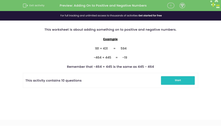# Add or Subtract Negative Numbers

In this worksheet, students will add or subtract negative numbers.Key stage:  KS 3

Curriculum topic:   Number

Curriculum subtopic:   Use Four Operations for All Numbers

Popular topics:   Subtraction worksheets, Addition worksheets

Difficulty level:#### Worksheet Overview

181  + 431 =  594

-464 + 445  = -19

Remember that -464 + 445 is the same as 445 - 464

Subtracting

420 - 421 = -1

-160 - 160 = -320

Think of a number line if it helps.  When you subtract, you move to the left to make a number more negative.

### What is EdPlace?

We're your National Curriculum aligned online education content provider helping each child succeed in English, maths and science from year 1 to GCSE. With an EdPlace account you’ll be able to track and measure progress, helping each child achieve their best. We build confidence and attainment by personalising each child’s learning at a level that suits them.

Get started